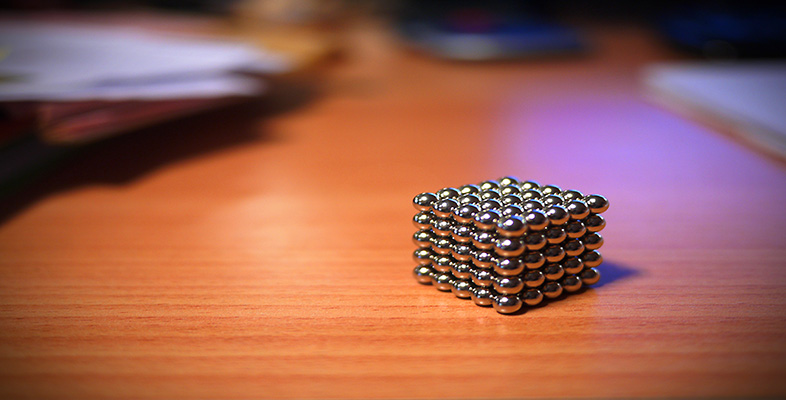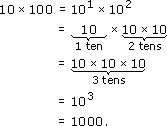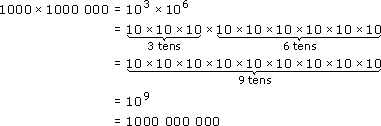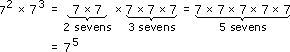Science, Maths & Technology

### Become an OU studentSquares, roots and powers

Start this free course now. Just create an account and sign in. Enrol and complete the course for a free statement of participation or digital badge if available.

# 2.3 Multiplying powers

Powers of ten can be used to investigate what happens when two powers of the same number are multiplied together. For example, consider multiplying 10 by 100:A billion is a thousand million. In terms of powers this is:so a billion is 109 or 1000 000 000.

(An American billion is a thousand million. In UK a billion used to be a million million, but now the American billion is the standard usage.)

Note: The number of zeros in the whole number power of ten is the same as the power.

In the above calculations, 101 × 102 = 103 and 103 × 106 = 109, and the way these have been worked out shows that, when you multiply numbers expressed as powers of ten, you add the powers.

Powers of 10 were used because they are easy to work with, but you can just as easily show that multiplying powers of the same number always means adding the powers. For example:so 72 × 73 = 72+3 = 75. Check this on your calculator!

To multiply two powers of the same number, add the powers.